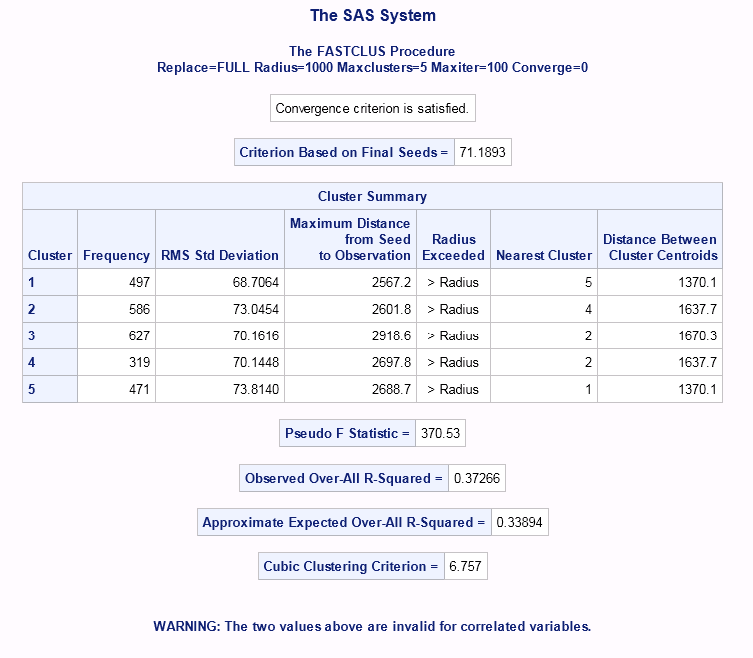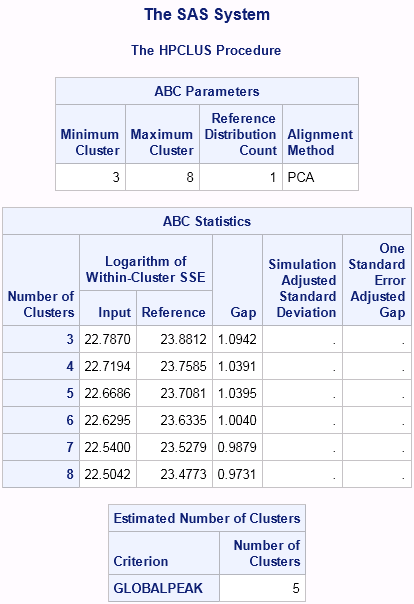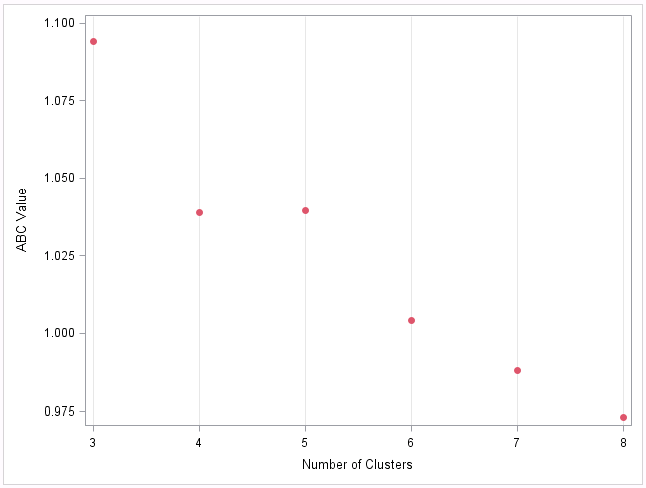## Tip: K-means clustering in SAS - comparing PROC FASTCLUS and PROC HPCLUS

Started ‎08-04-2014 by
Modified ‎11-04-2019 by
Views 91,772

SAS has many PROCs for performing different types of clustering tasks, but this tip will focus on using the well-known k-means algorithm ( http://en.wikipedia.org/wiki/K-means_clustering) as implemented by the FASTCLUS procedure in SAS/STAT® and the HPCLUS procedure in SAS® Enterprise Miner™. The k-means algorithm assigns clusters to observations in a way that minimizes the distance between observations and their assigned cluster centroids. This is done in an iterative approach by reassigning cluster membership and cluster centroids until the solution reaches a local optimum. While both procedures implement standard k-means, PROC FASTCLUS achieves fast convergence through non-random initialization, while PROC HPCLUS enables clustering of large data sets through multithreaded and distributed computing. The two procedures also differ in a few implementation details, as outlined below.

Standardization

Variables measured on different scales (such as age and income) should be standardized prior to clustering, so that the solution is not driven by variables measured on larger scales. PROC FASTCLUS does not have a standardization option, and you should use methods such as PROC STDIZE to standardize input data prior to running FASTCLUS. In contrast, PROC HPCLUS provides a STANDARDIZE option with range and z-score standardization.

Initialization

PROC FASTCLUS selects the first k complete (no missing values) observations that are RADIUS (default set to 0) apart from each other as initial cluster centroids. As such, the ordering of observations can affect initial centroids selection, and FASTCLUS is not recommended for data sets with fewer than 100 observations. You can use the REPLACE=RANDOM option to select pseudo-random centroids instead.

In Enterprise Miner 13.2, PROC HPCLUS provides only random initialization, using pseudo-random indices to select k complete observations as initial cluster centroids. You can specify the seed for the random number generator using the SEED= option, which can provide consistency across runs that use same number of nodes and threads.

Clustering Nominal variables

PROC FASTCLUS does not support the clustering of nominal variables. In Enterprise Miner 13.2, PROC HPCLUS uses the k-modes algorithm (http://shapeofdata.wordpress.com/2014/03/04/k-modes/), where cluster centroids are replaced by the modes of the nominal variables, for clustering nominal data sets. The DISTANCENOM option offers several choices for determining the distance between two nominal values, which can be simple matching distance or based on the occurrence frequency of the nominal value.

Prior to Enterprise Miner 13.2, PROC HPCLUS did not support clustering of nominal variables. Neither procedure currently supports the clustering of data with both numeric and nominal variables. The DISTANCE procedure can be used to obtain distance matrices of nominal variables for use in other clustering procedures such as CLUSTER or MODECLUS, as detailed in http://support.sas.com/kb/22/542.html.

Missing values

PROC FASTCLUS deals with observations with missing values by scaling the distance obtained from all non-missing variables. By default, PROC HPCLUS ignores observations with missing values. You can also select to impute missing values by using the IMPUTE option, which sets missing values to the means of numeric variables or the modes of nominal variables.

Determining the Number of Clusters k

PROC FASTCLUS considers k values less than or equal to the MAXCLUSTERS option, and it reports results for only a single k value, which is generally k=MAXCLUSTERS if MAXCLUSTERS is reasonably small. If the appropriate number of clusters is not known beforehand, you should try different values of MAXCLUSTERS and make a decision based on the output results. PROC FASTCLUS outputs several statistics that can be used to determine the best value of k, the interpretation of which will be discussed in an upcoming tip.

For numeric variables, PROC HPCLUS provides the convenient NOC=ABC option to auto-select the number of clusters k based on the aligned box criterion (ABC). For each k value from MINCLUSTERS (default to 2) to MAXCLUSTERS, ABC compares the within-cluster dispersion of the results to that of a simulated reference distribution, and selects a value of k where the within-cluster dispersions of the data results and the reference distribution differ greatly. The interpretation of the ABC value will also be discussed in further detail in an upcoming tip.

Scalability and Speed

PROC FASTCLUS has been used for enterprise scale problems for many years. It is a highly efficient but single-threaded procedure that decreases execution time by locating non-random cluster seeds.

PROC HPCLUS is one of many High-Performance Procedures in SAS Enterprise MIner 13.2. It is a multithreaded, distributed implementation of k-means that makes full use of multi-core processors and distributed computing environments. For example, PROC HPCLUS completed the extremely memory and computation-intensive task of assigning approximately 100 million observations with 13 variables to 1000 clusters in 46 minutes while executing on a 24 node Teradata appliance.

Examples

As an example, we will cluster the pixel values from handwritten digits taken from the MNIST database. Previous research has shown that clusters in the MNIST database are overlapping and non-spherical. To create easily interpretable results for this example, we will use 500 observations each from digits {0, 1, 2, 3, 4}. Note that standardization is not necessary since variables are measured in the same units.

Here are three ways to cluster our data&colon; 1) using purely FASTCLUS, 2) using purely HPCLUS, and 3) using a combination of both.

1) Using purely FASTCLUS.

If we don't know the number of expected clusters beforehand, we have to run PROC FASTCLUS multiple times with different k values. The macro below looks at k=3 to 8.

/* run fastclus for k from 3 to 8 */

%macro doFASTCLUS;

%do k= 3 %to 8;

proc fastclus

data= digits

out= fcOut

maxiter= 100

converge= 0          /* run to complete convergence */

radius= 100          /* look for initial centroids that are far apart */

maxclusters= &k

summary;

run;

%end;

%mend;

%doFASTCLUS

The summary output for each k includes four different statistics for determining the compactness and separation of the clustering results. The summary results for k=5 are shown below. Look for a future tip that discusses how to estimate the number of clusters using output statistics such as the Cubic Clustering Criterion and Pseudo F Statistic.2) Using purely HPCLUS

In HPCLUS, we can use the NOC= ABC option to auto-select the best k value between 3 and 8.

proc hpclus

data= digits

maxclusters= 8

maxiter= 100

seed= 54321                                 /* set seed for pseudo-random number generator */

NOC= ABC(B= 1 minclusters= 3 align= PCA);   /* select best k between 3 and 8 using ABC */

score out= OutScore;

input pixel:;                               /* input variables */

ods output ABCStats= ABC;                   /* save ABC criterion values for plotting */

run;

HPCLUS reports the best number of clusters in its output, along with the ABC gap values for each k value. The interpretation of ABC values will be discussed in detail in a future tip as well.We can view a plot of the ABC values for each k using the following code:

/* view ABC (used to determine best k) */

proc sgplot

data= ABC;

scatter x= K y= Gap / markerattrs= (color= 'STPK' symbol= 'circleFilled');

xaxis grid integer values= (3 to 8 by 1);

yaxis label= 'ABC Value';

run;

The slight peak at k=5 indicates that the best estimate for number of clusters is 5, which is expected for our data set of handwritten digits between 0-4.3) Combining FASTCLUS and HPCLUS.

Alternatively, we can combine the strengths of FASTCLUS and HPCLUS by first using HPCLUS to estimate the number of clusters k, then using FASTCLUS to obtain well-separated clusters through its non-random initialization.

/* run HPCLUS to auto-select best k value */

proc hpclus

data= digits

maxclusters= 8

maxiter= 100

seed= 54321

NOC= ABC(B= 1 minclusters= 3 align= PCA);   /* select best k between 3 and 8 using ABC */

input pixel:;

ods output ABCResults= k;                   /* save k value selected by ABC */

run;

/* get selected k into a macro var */

data _null_;

set k;

call symput('k', strip(k));

run;

%put k= &k.;

/* now run FASTCLUS to find k nice clusters from non-random seeds */

proc fastclus

data= digits

out= fcOut

maxiter= 100

converge= 0

radius= 100          /* look for initial centroids that are far apart */

maxclusters= &k    /* k found by hpclus */

summary;

run;

Summary

PROC FASTCLUS is suitable for small to medium-sized data.  It provides non-random initialization which often leads to more well-separated clusters, and it gives several statistics indicating the goodness of the clustering results. If you do not know what the number of clusters k should be beforehand, you would have to run FASTCLUS with different values of k to manually determine the best k.

HPCLUS is intended for running computationally demanding clustering tasks on distributed systems. It can automatically select the best k value within a specified range, but only supports random initialization.

See SAS/STAT 9.2 User's Guide Introduction to Cluster Procedures (https://support.sas.com/documentation/cdl/en/statugclustering/61759/PDF/default/statugclustering.pdf) for information on the many different clustering procedures in SAS. This document covers procedures from SAS/STAT, including CLUSTER, FASTCLUS, MODECLUS, VARCLUS, and TREE.

See Usage Note 22542: Clustering binary, ordinal, or nominal data (http://support.sas.com/kb/22/542.html)

Video tutorial

The tutorial below by SAS' @CatTruxillo walks you through two ways to do k-means clustering in SAS Visual Statistics and SAS Studio. Besides PROC FASTCLUS, described above, there are other ways to perform k-means clustering in SAS: you can write a program in PROC KCLUS, PROC CAS, Python, or R. You can point and click in SAS Visual Statistics, Enterprise Guide, Enterprise Miner, JMP, Model Studio, and SAS Studio.

Version history
Last update:
‎11-04-2019 04:02 PM
Updated by:Contributors
Article Labels
Article Tags